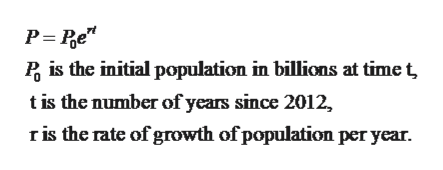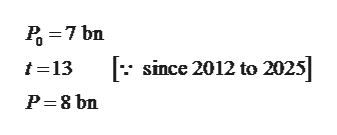In 2012, the worlds population was 7 billion and the population was projected to reach 8 billion by the year 2025. What was the annual growth rate projected? Round to four decimals.

Question

In 2012, the worlds population was 7 billion and the population was projected to reach 8 billion by the year 2025. What was the annual growth rate projected? Round to four decimals.

Step 1

Given:

In 2012, the world’s population was 7 billion and the population was projected to reach 8 billion by the year 2025. What was the annual growth rate projected?  Round to four decimals.

Step 2

Since the population growth equation is given by:help_outlineImage TranscriptionclosePis the initial population in billions at time t tis the number of years since 2012, r is the rate of growth of population per year. fullscreen
Step 3

So In this c...help_outlineImage Transcriptionclose7 bn since 2012 to 2025] t-13 P 8 bn fullscreen

Want to see the full answer?

See Solution

Want to see this answer and more?

Our solutions are written by experts, many with advanced degrees, and available 24/7

See Solution
Tagged in

Other